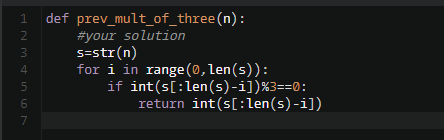# 【每日一题0830】与3为伍霍格沃兹魔法学院流传着一个关于数字3的游戏，规则是输入一个数字`n` ，如果n能被3整除则直接返回`n` 。如果不能，那么尝试每次剔除掉一个末尾数字并检查剩下的数字能否被3整除，如果可以就返回剩下的数字，否则返回`None` 。请编写一个python3函数来实现它吧。

``````def prev_mult_of_three(n: int):
pass

assert prev_mult_of_three(36) == 36
assert prev_mult_of_three(1) == None
assert prev_mult_of_three(1244) == 12
assert prev_mult_of_three(952406) == 9
``````
``````def prev_mult_of_three(n: int):
return None if len(str(n)) == 1 and n % 3 else n if n % 3 == 0 else prev_mult_of_three(int(str(n)[:-1]))

assert prev_mult_of_three(36) == 36
assert prev_mult_of_three(1) == None
assert prev_mult_of_three(1244) == 12
assert prev_mult_of_three(952406) == 9
``````
``````def prev_mult_of_three(n):
if n % 3 == 0:
return n

s = str(n)[::-1]

for i in range(1,len(s)):
if int(s[i:]) % 3 == 0:
return int(s[i:][::-1])
``````

def prev_mult_of_three(n: int):
if n%3==0:
return n
else:
s=str(n)
s=s[::-1]
for i in range(0,len(s)):
if int(s[i:])%3==0:
return int(s[i:][::-1])

``````  public Integer prevMultOfThree(Integer integer) {
// 霍格沃兹魔法学院流传着一个关于数字3的游戏，规则是输入一个数字n ，如果n能被3整除则直接返回n 。如果不能，那么尝试每次剔除掉一个末尾数字并检查剩下的数字能否被3整除，如果可以就返回剩下的数字，否则返回None 。请编写一个python3函数来实现它吧。
// 示例：
// 输入：36 ，输出：36
// 输入：1 ，输出：0
// 输入：952406 ，输出：9
return integer % 3 == 0 ? integer : prevMultOfThree(integer / 10);
}

@Test
public void prevMultOfThreeTest() {
assert prevMultOfThree(36) == 36;
assert prevMultOfThree(1244) == 12;
assert prevMultOfThree(952406) == 9;
assert prevMultOfThree(1) == 0;
}
````````````def prev_mult_of_three(n: int):
# 先把一位数且不能整除3的return None
if len(str(n)) == 1 and n % 3 != 0:
return None

# 原数能被3整除，return n
if n % 3 == 0:
return n

# 原数不能被3整除，删掉最后一位，再次判断，直到能被整除或不能整除
for i in range(len(str(n))):
n = str(n)[:-1]
# 加这个判断的作用防止删掉剩最后一位，且不能被整除，会报ValueError错
if len(n) == 1 and int(n) % 3 != 0:
return None

elif int(n) % 3 == 0:
return int(n)

assert prev_mult_of_three(36) == 36
assert prev_mult_of_three(1244) == 12
assert prev_mult_of_three(952406) == 9
assert prev_mult_of_three(9524061) == 9524061
assert prev_mult_of_three(1) == None
assert prev_mult_of_three(10) == None
assert prev_mult_of_three(100) == None
assert prev_mult_of_three(112) == None
assert prev_mult_of_three(1000) == None
assert prev_mult_of_three(10000) == None
assert prev_mult_of_three(1000000) == None
``````

@1286175038_5382 Emmm如果是按照从后往前的话，你这样做已经比较精简了，我想不出更好的办法。我当时选择先逆序再求和就是为了偷懒